# 浅谈算法和数据结构十一 哈希表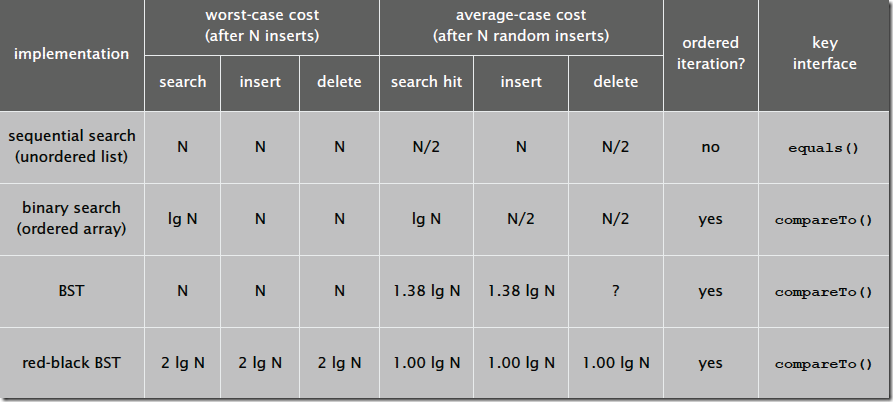# 什么是哈希表

1. 使用哈希函数将被查找的键转换为数组的索引。在理想的情况下，不同的键会被转换为不同的索引值，但是在有些情况下我们需要处理多个键被哈希到同一个索引值的情况。所以哈希查找的第二个步骤就是处理冲突
2. 处理哈希碰撞冲突。有很多处理哈希碰撞冲突的方法，本文后面会介绍拉链法和线性探测法。

# 哈希函数

## 2. 字符串

``````public int GetHashCode(string str)
{
char[] s = str.ToCharArray();
int hash = 0;
for (int i = 0; i < s.Length; i++)
{
hash = s[i] + (31 * hash);
}
return hash;
}``````

h = s · 31L–1 + … + s[L – 3] · 312 + s[L – 2] · 311 + s[L – 1] · 310

``````public int GetHashCode(string str)
{
char[] s = str.ToCharArray();
int hash = 0;
int skip = Math.Max(1, s.Length / 8);
for (int i = 0; i < s.Length; i+=skip)
{
hash = s[i] + (31 * hash);
}
return hash;
}``````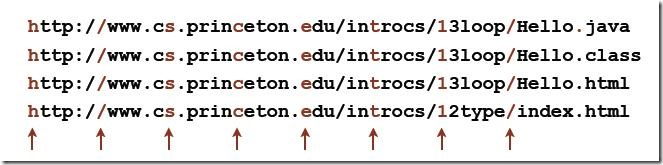# 避免哈希冲突

## 拉链法 (Separate chaining with linked lists)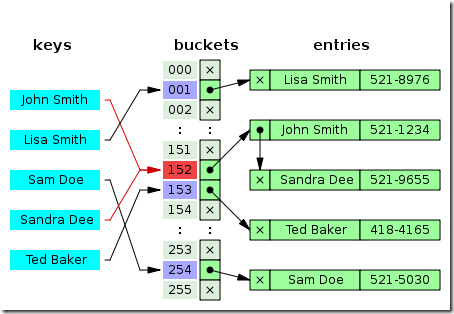``````public class SeperateChainingHashSet<TKey, TValue> : SymbolTables<TKey, TValue> where TKey : IComparable<TKey>, IEquatable<TKey>
{
private int M;//散列表大小
private SequentSearchSymbolTable<TKey, TValue>[] st;//

public SeperateChainingHashSet()
: this(997)
{

}

public SeperateChainingHashSet(int m)
{
this.M = m;
st = new SequentSearchSymbolTable<TKey, TValue>[m];
for (int i = 0; i < m; i++)
{
st[i] = new SequentSearchSymbolTable<TKey, TValue>();
}
}

private int hash(TKey key)
{
return (key.GetHashCode() & 0x7fffffff) % M;
}

public override TValue Get(TKey key)
{
return st[hash(key)].Get(key);
}

public override void Put(TKey key, TValue value)
{
st[hash(key)].Put(key, value);
}

}``````

• Get方法来获取指定key的Value值，我们首先通过hash方法来找到key对应的索引值，即找到SequentSearchSymbolTable数组中存储该元素的查找表，然后调用查找表的Get方法，根据key找到对应的Value。
• Put方法用来存储键值对，首先通过hash方法找到改key对应的哈希值，然后找到SequentSearchSymbolTable数组中存储该元素的查找表，然后调用查找表的Put方法，将键值对存储起来。
• hash方法来计算key的哈希值， 这里首先通过取与&操作，将符号位去除，然后采用除留余数法将key应到到0-M-1的范围，这也是我们的查找表数组索引的范围。

## 线性探测法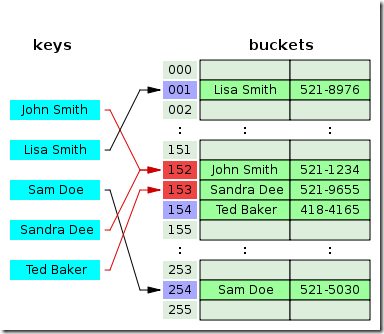1. 命中，该位置的键和被查找的键相同
2. 未命中，键为空
3. 继续查找，该位置和键被查找的键不同。

``````public class LinearProbingHashSet<TKey, TValue> : SymbolTables<TKey, TValue> where TKey : IComparable<TKey>, IEquatable<TKey>
{
private int N;//符号表中键值对的总数
private int M = 16;//线性探测表的大小
private TKey[] keys;
private TValue[] values;

public LinearProbingHashSet()
{
keys = new TKey[M];
values = new TValue[M];
}

private int hash(TKey key)
{
return (key.GetHashCode() & 0xFFFFFFF) % M;
}

public override TValue Get(TKey key)
{
for (int i = hash(key); keys[i] != null; i = (i + 1) % M)
{
if (key.Equals(keys[i])) { return values[i]; }
}
return default(TValue);
}

public override void Put(TKey key, TValue value)
{
int hashCode = hash(key);
for (int i = hashCode; keys[i] != null; i = (i + 1) % M)
{
if (keys[i].Equals(key))//如果和已有的key相等，则用新值覆盖
{
values[i] = value;
return;
}
//插入
keys[i] = key;
values[i] = value;
}
}
}``````

# 哈希碰撞攻击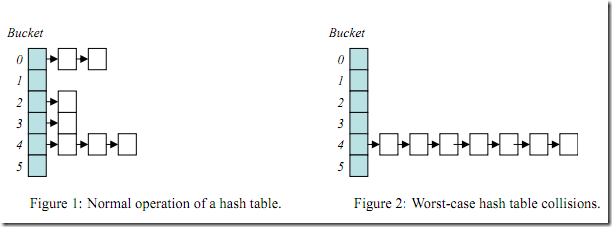``````[ReliabilityContract(Consistency.WillNotCorruptState, Cer.MayFail), SecuritySafeCritical, __DynamicallyInvokable]
public override unsafe int GetHashCode()
{
if (HashHelpers.s_UseRandomizedStringHashing)
{
return InternalMarvin32HashString(this, this.Length, 0L);
}
fixed (char* str = ((char*) this))
{
char* chPtr = str;
int num = 0x15051505;
int num2 = num;
int* numPtr = (int*) chPtr;
int length = this.Length;
while (length > 2)
{
num = (((num << 5) + num) + (num >> 0x1b)) ^ numPtr;
num2 = (((num2 << 5) + num2) + (num2 >> 0x1b)) ^ numPtr;
numPtr += 2;
length -= 4;
}
if (length > 0)
{
num = (((num << 5) + num) + (num >> 0x1b)) ^ numPtr;
}
return (num + (num2 * 0x5d588b65));
}
}``````

# .NET中哈希的实现

``````public Dictionary(int capacity, IEqualityComparer<TKey> comparer) {
if (capacity < 0) ThrowHelper.ThrowArgumentOutOfRangeException(ExceptionArgument.capacity);
if (capacity > 0) Initialize(capacity);
this.comparer = comparer ?? EqualityComparer<TKey>.Default;
}``````

``````private void Initialize(int capacity) {
int size = HashHelpers.GetPrime(capacity);
buckets = new int[size];
for (int i = 0; i < buckets.Length; i++) buckets[i] = -1;
entries = new Entry[size];
freeList = -1;
}``````

``````private void Insert(TKey key, TValue value, bool add)
{
if( key == null ) {
ThrowHelper.ThrowArgumentNullException(ExceptionArgument.key);
}

if (buckets == null) Initialize(0);
int hashCode = comparer.GetHashCode(key) & 0x7FFFFFFF;
int targetBucket = hashCode % buckets.Length;

#if FEATURE_RANDOMIZED_STRING_HASHING
int collisionCount = 0;
#endif

for (int i = buckets[targetBucket]; i >= 0; i = entries[i].next) {
if (entries[i].hashCode == hashCode && comparer.Equals(entries[i].key, key)) {
}
entries[i].value = value;
version++;
return;
}

#if FEATURE_RANDOMIZED_STRING_HASHING
collisionCount++;
#endif
}
int index;
if (freeCount > 0) {
index = freeList;
freeList = entries[index].next;
freeCount--;
}
else {
if (count == entries.Length)
{
Resize();
targetBucket = hashCode % buckets.Length;
}
index = count;
count++;
}

entries[index].hashCode = hashCode;
entries[index].next = buckets[targetBucket];
entries[index].key = key;
entries[index].value = value;
buckets[targetBucket] = index;
version++;

#if FEATURE_RANDOMIZED_STRING_HASHING
if(collisionCount > HashHelpers.HashCollisionThreshold && HashHelpers.IsWellKnownEqualityComparer(comparer))
{
comparer = (IEqualityComparer<TKey>) HashHelpers.GetRandomizedEqualityComparer(comparer);
Resize(entries.Length, true);
}
#endif

}``````

# 总结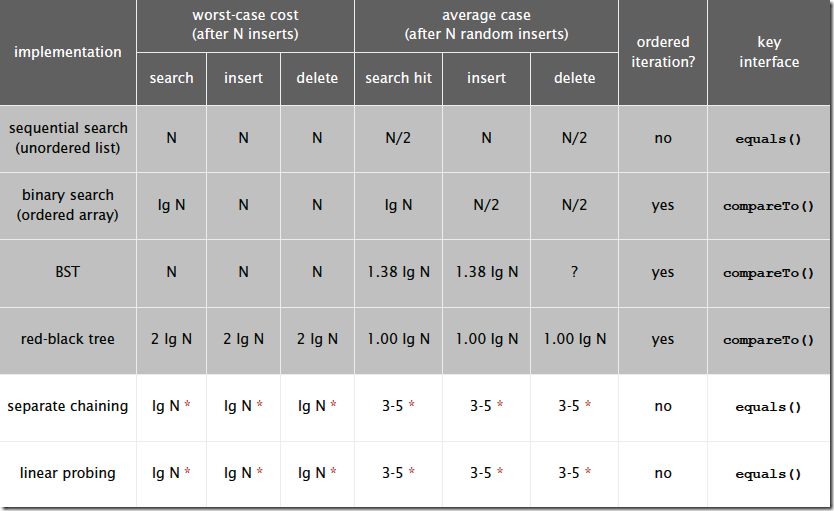Do you have any suggestions for improvement?